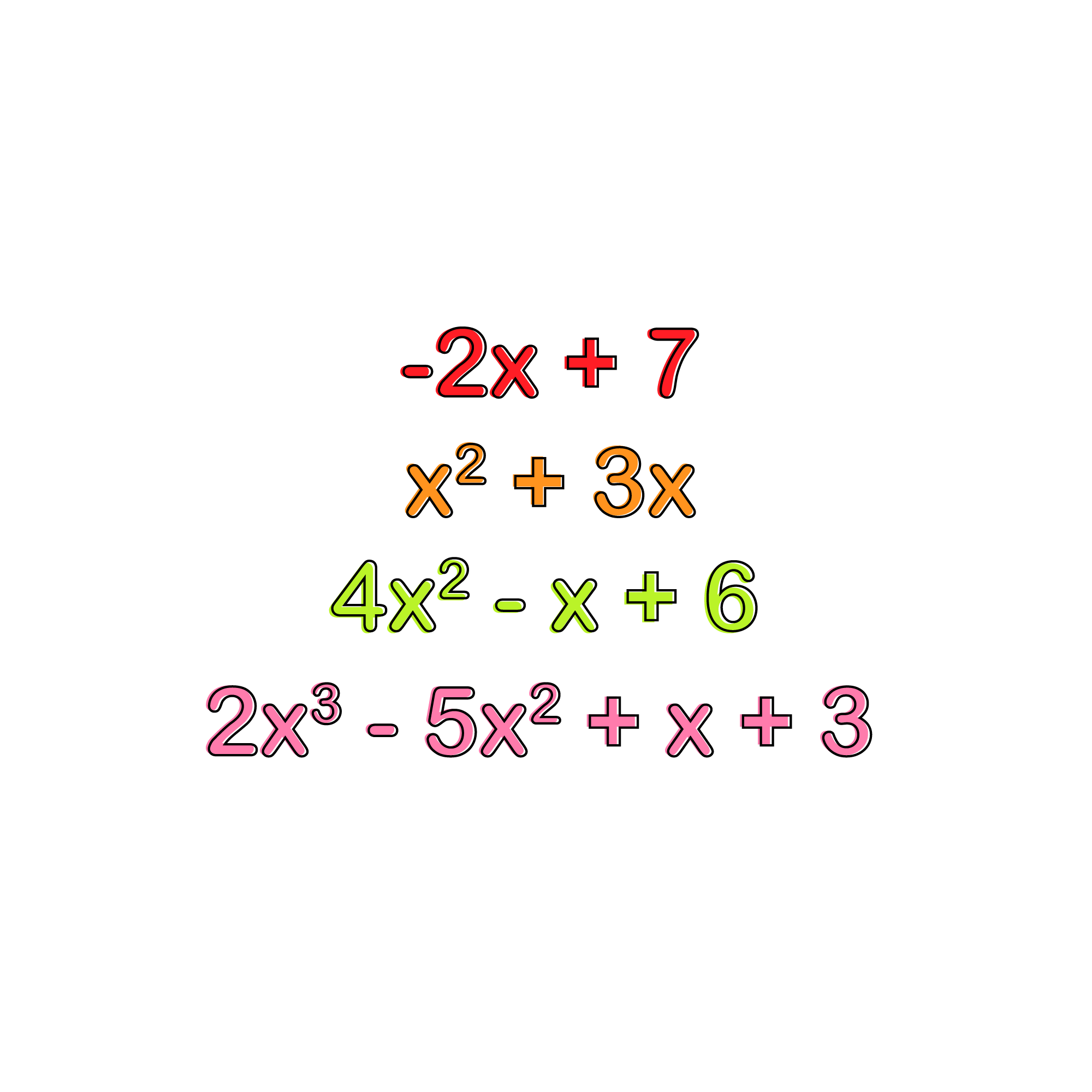SAT Math Algebra and Functions

## Polynomials

#### A polynomial is an algebraic expression comprised of more than two terms, usually of like bases and different powers. Polynomials frequently include integer terms as well.

$$3x^{2} + 2x - 4$$
$$x^{1} - 6x^{2} + x - 0$$
$$7x^{3} + x^{2} + x + 1$$

The key to adding polynomials is identifying the various like bases and then proceeding to add their coefficients. For instance, let’s add the following polynomials: $$(x^{2} + 2x - 1) + (3x - 2x^{4} + 4)$$

Drop the parentheses, making the expression: $$x^{2} + 2x - 1 + 3x - 2x + 4$$

Next, identify the like terms. In this particular case, the like terms include $$x^{2}$$ , $$x$$, and the integer term.

Add the coefficient of each like base together to create the new term: $$-x^{2} + 5x + 3$$

## 2. Multiplying Polynomials

When it comes to multiplying polynomials, the most important thing to remember is that each term from one polynomial will need to be multiplied with each term from the other polynomials.

Such an example of polynomial multiplication is $$(Y + 3 - Y^{2})(2Y - 1 + 2Y^{2})$$

#### First, multiply the first term from the first polynomial with each term in the second polynomial; this results in

Y x 2Y = $$2Y^{2}$$
Y x -1 = -Y
Y x $$2Y^{2} = 2Y^{3}$$

#### Next, multiply the second term in the first polynomial with each term in the second polynomial; this results in

3 x 2Y = 6Y
3 x -1 = -3
3 x $$2Y^{2} = 6Y^{2}$$

#### Then, multiply the third term in the first polynomial with each term in the second polynomial; this results in

$$-Y^{2}$$ x 2Y = $$-2Y^{3}$$
$$-Y^{2}$$ x -1 = $$Y^{2}$$
-Y x $$2Y{2}$$ = $$-2Y^{4}$$

#### From here, we combine all terms into a polynomial,

$$2Y^{2} - Y + 2Y^{3} + 6Y - 3 + 6Y^{2} - 2Y^{3} + Y^{2} - 2Y^{4}$$

#### Now, combine like base terms to simplify further.

$$-2Y^{4} + 9Y^{2} + 5Y - Y - 3$$

## 3. Dividing Polynomials

When dividing polynomials, it is important to identify like bases and use the rule of exponents to simplify the terms which contain variables. Similarly to multiplying polynomials, when dividing polynomials each term in the dividend must be divided by each term in the divisor.

#### One way to accomplish division with polynomials is to perform long division, which is also known as synthetic division. An example of using long division with polynomials is as follows:

$$(12x^{2} + 2x +4)$$ ÷ (2x + 1)

Dividend: $$12x^{2}$$ + 2x + 4
Divisor:
2x + 1

Performing this division will result in finding the quotient: $$2x + 1\sqrt{12x^{2} + 2x + 4}$$

In order to start dividing, look at the first term in the divisor and the first term in the dividend. In this particular case, 2x goes into $$12x^{2}$$ , 6 times. In other words 2x x 6x = $$12x^{2}$$ . Now multiply 6x to each term in the divisor.

From here, subtract the multiplied-out terms from the terms in the dividend.

Now, look at the second term in the dividend and see how many times the first term in the divisor can go into it. In this particular case, 2x goes into 2x one time. Thus we have:

From here, multiply the second terms of the quotient with the divisor and subtract from the dividend.

Since there are no terms left to subtract from, we place the remainder (-6x + 3) over the divisor in the quotient to create the final polynomial: $$6x + 1 + \frac{-6x + 3}{2x + 1}$$